# Maharashtra Board 12th Commerce Maths Solutions Chapter 8 Probability Distributions Miscellaneous Exercise 8

Balbharati Maharashtra State Board 12th Commerce Maths Solution Book Pdf Chapter 8 Probability Distributions Miscellaneous Exercise 8 Questions and Answers.

## Maharashtra State Board 12th Commerce Maths Solutions Chapter 8 Probability Distributions Miscellaneous Exercise 8

(I) Choose the correct alternative.

Question 1.
F(x) is c.d.f. of discreter r.v. X whose p.m.f. is given by P(x) = $$k\left(\begin{array}{l} 4 \\ x \end{array}\right)$$, for x = 0, 1, 2, 3, 4 & P(x) = 0 otherwise then F(5) = __________
(a) $$\frac{1}{16}$$
(b) $$\frac{1}{8}$$
(c) $$\frac{1}{4}$$
(d) 1
(d) 1

Question 2.
F(x) is c.d.f. of discrete r.v. X whose distribution is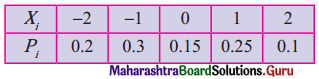then F(-3) = __________
(a) 0
(b) 1
(c) 0.2
(d) 0.15
(a) 0Question 3.
X : number obtained on uppermost face when a fair die is thrown then E(X) = __________
(a) 3.0
(b) 3.5
(c) 4.0
(d) 4.5
(b) 3.5

Question 4.
If p.m.f. of r.v. X is given below.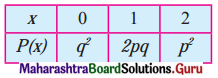then Var(X) = __________
(a) p2
(b) q2
(c) pq
(d) 2pq
(d) 2pq

Question 5.
The expected value of the sum of two numbers obtained when two fair dice are rolled is __________
(a) 5
(b) 6
(c) 7
(d) 8
(c) 7

Question 6.
Given p.d.f. of a continuous r.v. X as
f(x) = $$\frac{x^{2}}{3}$$ for -1 < x < 2
= 0 otherwise then F(1) =
(a) $$\frac{1}{9}$$
(b) $$\frac{2}{9}$$
(c) $$\frac{3}{9}$$
(d) $$\frac{4}{9}$$
(b) $$\frac{2}{9}$$

Question 7.
X is r.v. with p.d.f.
f(x) = $$\frac{k}{\sqrt{x}}$$, 0 < x < 4
= 0 otherwise then E(X) = __________
(a) $$\frac{1}{3}$$
(b) $$\frac{4}{3}$$
(c) $$\frac{2}{3}$$
(d) 1
(b) $$\frac{4}{3}$$

Question 8.
If X follows B(20, $$\frac{1}{10}$$) then E(X) = __________
(a) 2
(b) 5
(c) 4
(d) 3
(a) 2

Question 9.
If E(X) = m and Var(X) = m then X follows __________
(a) Binomial distribution
(b) Possion distribution
(c) Normal distribution
(d) none of the above
(b) Possion distributionQuestion 10.
If E(X) > Var(X) then X follows __________
(a) Binomial distribution
(b) Possion distribution
(c) Normal distribution
(d) none of the above
(a) Binomial distribution

(II) Fill in the blanks.

Question 1.
The values of discrete r.v. are generally obtained by __________
counting

Question 2.
The values of continuous r.v. are generally obtained by __________
measurement

Question 3.
If X is dicrete random variable takes the values x1, x2, x3, …… xn then $$\sum_{i=1}^{n} p\left(x_{i}\right)$$ = __________
1

Question 4.
If f(x) is distribution function of discrete r.v. X with p.m.f. p(x) = $$\frac{x-1}{3}$$ for x = 1, 2, 3, and p(x) = 0 otherwise then F(4) = __________
1

Question 5.
If f(x) is distribution function of discrete r.v. X with p.m.f. p(x) = $$k\left(\begin{array}{l} 4 \\ x \end{array}\right)$$ for x = 0, 1, 2, 3, 4, and p(x) = 0 otherwise then F(-1) = __________
0Question 6.
E(X) is considered to be __________ of the probability distribution of X.
centre of gravity

Question 7.
If X is continuous r.v. and f(xi) = P(X ≤ xi) = $$\int_{-\infty}^{x_{i}} f(x) d x$$ then f(x) is called __________
Cumulative Distribution Function

Question 8.
In Binomial distribution probability of success ________ from trial to trial.
remains constant/independent

Question 9.
In Binomial distribution, if n is very large and probability success of p is very small such that np = m (constant) then ________ distribution is applied.
Possion

(III) State whether each of the following is True or False.

Question 1.
If P(X = x) = $$k\left(\begin{array}{l} 4 \\ x \end{array}\right)$$ for x = 0, 1, 2, 3, 4, then F(5) = $$\frac{1}{4}$$ when f(x) is c.d.f.
False

Question 2.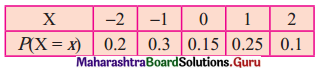If F(x) is c.d.f. of discrete r.v. X then F(-3) = 0.
True

Question 3.
X is the number obtained on the uppermost face when a die is thrown the E(X) = 3.5.
TrueQuestion 4.
If p.m.f. of discrete r.v.X is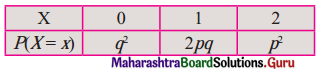then E(X) = 2p.
True

Question 5.
The p.m.f. of a r.v. X is p(x) = $$\frac{2 x}{n(n+1)}$$, x = 1, 2,……n
= 0 otherwise,
Then E(X) = $$\frac{2 n+1}{3}$$
True

Question 6.
If f(x) = kx (1 – x) for 0 < x < 1
= 0 otherwise then k = 12
False

Question 7.
If X ~ B(n, p) and n = 6 and P(X = 4) = P(X = 2) then p = $$\frac{1}{2}$$.
True

Question 8.
If r.v. X assumes values 1, 2, 3,………, n with equal probabilities then E(X) = $$\frac{(n+1)}{2}$$
True

Question 9.
If r.v. X assumes the values 1, 2, 3,………, 9 with equal probabilities, E(X) = 5.
True

(IV) Solve the following problems.

Part – I

Question 1.
Identify the random variable as discrete or continuous in each of the following. Identify its range if it is discrete.
(i) An economist is interested in knowing the number of unemployed graduates in the town with a population of 1 lakh.
Solution:
X = No. of unemployed graduates in a town.
∵ The population of the town is 1 lakh
∴ X takes finite values
∴ X is a Discrete Random Variable
∴ Range of = {0, 1, 2, 4, …. 1,00,000}

(ii) Amount of syrup prescribed by a physician.
Solution:
X : Amount of syrup prescribed.
∴ X Takes infinite values
∴ X is a Continuous Random Variable.

(iii) A person on a high protein diet is interested in the weight gained in a week.
Solution:
X : Gain in weight in a week.
X takes infinite values
∴ X is a Continuous Random Variable.(iv) Twelve of 20 white rats available for an experiment are male. A scientist randomly selects 5 rats and counts the number of female rats among them.
Solution:
X : No. of female rats selected
X takes finite values.
∴ X is a Discrete Random Variable.
Range of X = {0, 1, 2, 3, 4, 5}

(v) A highway safety group is interested in the speed (km/hrs) of a car at a checkpoint.
Solution:
X : Speed of car in km/hr
X takes infinite values
∴ X is a Continuous Random Variable.

Question 2.
The probability distribution of a discrete r.v. X is as follows.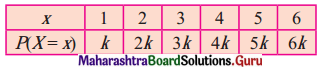(i) Determine the value of k.
(ii) Find P(X ≤ 4), P(2 < X < 4), P(X ≥ 3).
Solution:
(i) Assuming that the given distribution is a p.m.f. of X
∴ Each P(X = x) ≥ 0 for x = 1, 2, 3, 4, 5, 6
k ≥ 0
ΣP(X = x) = 1 and
k + 2k + 3k + 4k + 5k + 6k = 1
∴ 21k = 1 ∴ k = $$\frac{1}{21}$$

(ii) P(X ≤ 4) = 1 – P(X > 4)
= 1 – [P(X = 5) + P(X = 6)]
= 1 – $\frac{5}{21}+\frac{6}{21}$
= 1 – $$\frac{11}{21}$$
= $$\frac{10}{21}$$
P(2 < X < 6) = p(3) + p(4) + p(5)
= 3k + 4k + 5k
= $$\frac{3}{21}+\frac{4}{21}+\frac{5}{21}$$
= $$\frac{12}{21}$$
= $$\frac{4}{7}$$

(iii) P(X ≥ 3) = p(3) + p(4) + p(5) + p(6)
= 3k + 4k + 5k + 6k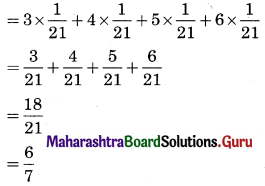Question 3.
Following is the probability distribution of an r.v. X.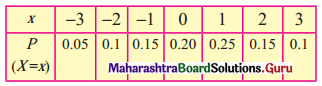Find the probability that
(i) X is positive.
(ii) X is non-negative.
(iii) X is odd.
(iv) X is even.
Solution:
(i) P(X is positive)
P(X = 0) = p(1) + p(2) + p(3)
= 0.25 + 0.15 + 0.10
= 0.50

(ii) P(X is non-negative)
P(X ≥ 0) = p(0) + p(1) + p(2) + p(3)
= 0.20 + 0.25 + 0.15 + 0.10
= 0.70

(iii) P(X is odd)
P(X = -3, -1, 1, 3)
= p(- 3) +p(-1) + p(1) + p(3)
= 0.05 + 0.15 + 0.25 + 0.10
= 0.55

(iv) P(X is even)
= 1 – P(X is odd)
= 1 – 0.55
= 0.45Question 4.
The p.m.f of a r.v. X is given by
$$P(X=x)= \begin{cases}\left(\begin{array}{l} 5 \\ x \end{array}\right) \frac{1}{2^{5}}, & x=0,1,2,3,4,5 . \\ 0 & \text { otherwise }\end{cases}$$
Show that P(X ≤ 2) = P(X ≥ 3).
Solution:
For x = 0, 1, 2, 3, 4, 5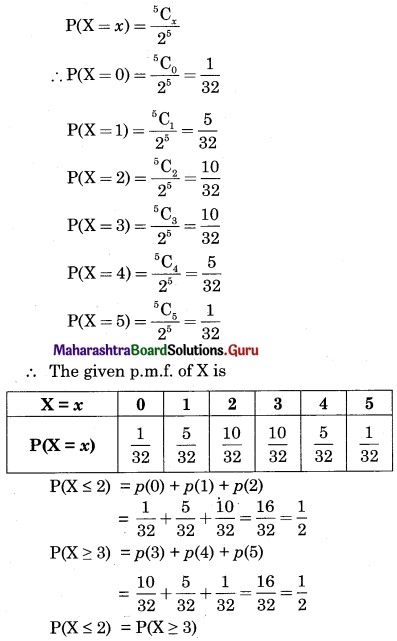Question 5.
In the following probability distribution of an r.v. X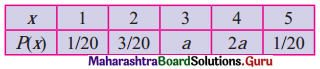Find a and obtain the c.d.f. of X.
Solution:
Given distribution is p.m.f. of r.v. X
ΣP(X = x) = 1
∴ p(1) + p(2) + p(3) + p(4) + p(5) = 1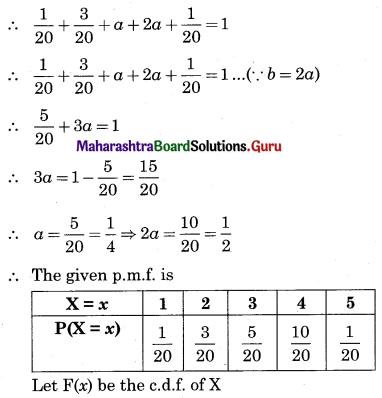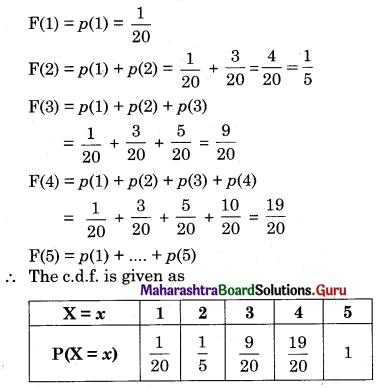Question 6.
A fair coin is tossed 4 times. Let X denote the number of heads obtained. Identify the probability distribution of X and state the formula for p.m.f. of X.
Solution:
A fair coin is tossed 4 times
∴ Sample space contains 16 outcomes
Let X = Number of heads obtained
∴ X takes the values x = 0, 1, 2, 3, 4.
∴ The number of heads obtained in a toss is an even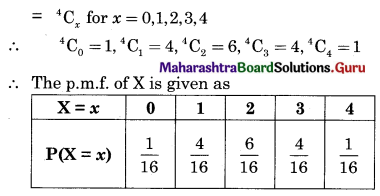Question 7.
Find the probability of the number of successes in two tosses of a die, where success is defined as (i) number greater than 4 (ii) six appearing in at least one toss.
Solution:
S : A die is tossed two times
S = {(1, 1), (1, 2), (1, 3), (1, 4), (1, 5), (1, 6), (2, 1), (2, 2), (2, 3), (2, 4), (2, 5), (2, 6), (3, 1), (3, 2), (3, 3), (3, 4), (3, 5), (3, 6), (4, 1), (4, 2), (4, 3), (4, 4), (4, 5), (4, 6), (5, 1), (5, 2), (5, 3), (5, 4), (5, 5), (5, 6), (6, 1), (6, 2), (6, 3), (6, 4), (6, 5), (6, 6)}
n(S) = 36
(i) X : No. is greater than 4
Range of X = {0, 1, 2}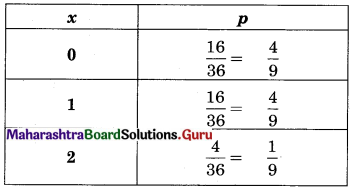(ii) X : Six appears on aleast one die.
Range of X = {0, 1, 2}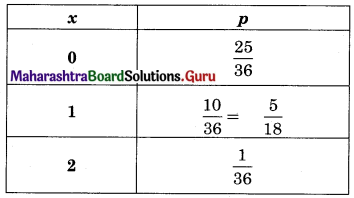Question 8.
A random variable X has the following probability distribution.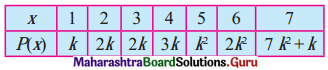Determine (i) k, (ii) P(X < 3), (iii) P(X > 6), (iv) P(0 < X < 3).
Solution:
(i) It is a p.m.f. of r.v. X
Σp(x) = 1
p(1) + p(2) + p(3) + p(4) + p(5) + p(6) + p(7) = 1
k + 2k + 2k + 3k + k2 + 2k2 + 7k2 + k = 1
9k + 10k2 = 1
10k2 + 9k – 1 = 0
10k2 +10k – k – 1 = 0
∴ 10k(k + 1) – 1(k + 1) = 0
∴ (10k – 1) (k + 1) = 0
∴ 10k – 1 = 0r k + 1 = 0
∴ k = $$\frac{1}{10}$$ or k = -1
k = -1 is not accepted, p(x) ≥ 0, ∀ x ∈ R
∴ k = $$\frac{1}{10}$$(ii) P(X < 3) = p(1) + p(2)
= k + 2k
= 3k
= 3 × $$\frac{1}{10}$$
= $$\frac{3}{10}$$

(iii) P(X > 6) = p(7)
= 7k2 + k
= $$7\left(\frac{1}{10}\right)^{2}+\frac{1}{10}$$
= $$\frac{7}{100}+\frac{1}{10}$$
= $$\frac{17}{100}$$

(iv) P(0 < X < 3) = p(1) + p(2)
= k + 2k
= 3k
= 3 × $$\frac{1}{10}$$
= $$\frac{3}{10}$$

Question 9.
The following is the c.d.f. of a r.v. X.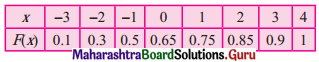Find the probability distribution of X and P(-1 ≤ X ≤ 2).
Solution: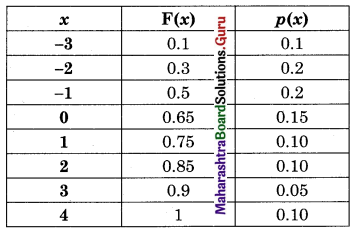P(-1 ≤ X ≤ 2) = p(-1) + p(0) + p(1) + p(2)
= 0.2 + 0.15 + 0.10 + 0.10
= 0.55

Question 10.
Find the expected value and variance of the r.v. X if its probability distribution is as follows.
(i)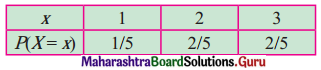Solution: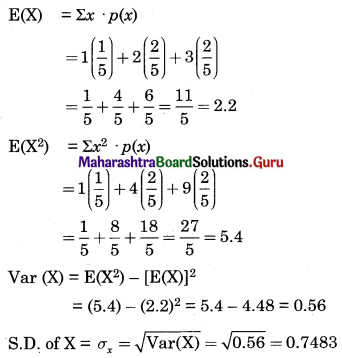(ii)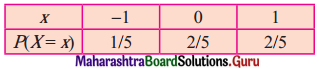Solution:
E(X) = Σx . p(x)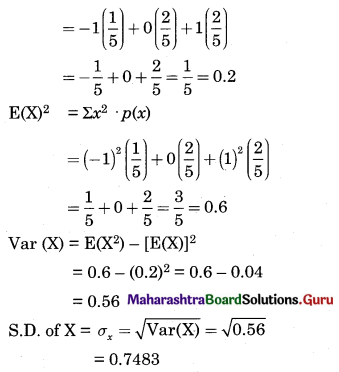(iii)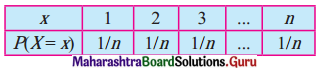Solution: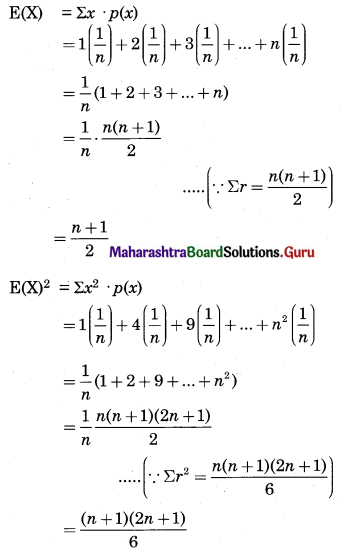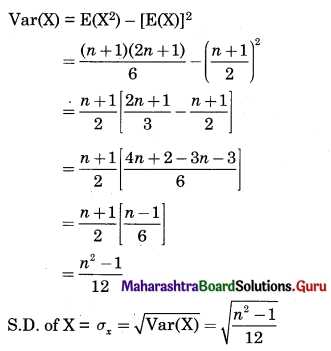(iv)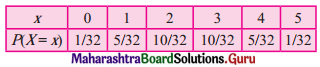Solution: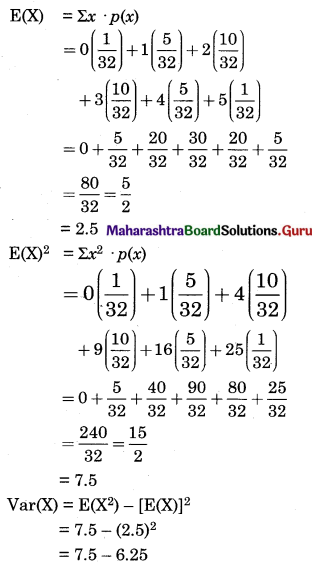= 1.25
S.D. of X = σx = √Var(X)
= √1.25
= 1.118

Question 11.
A player tosses two coins. He wins ₹ 10 if 2 heads appear, ₹ 5 if 1 head appears, and ₹ 2 if no head appears. Find the expected value and variance of the winning amount.
Solution:
S : Two fair coin are tossed
S = {HH, HT, TT, TH}
n(S) = 4
∴ Range of X = {0, 1, 2}
∴ Let Y = amount received corresponds to values of X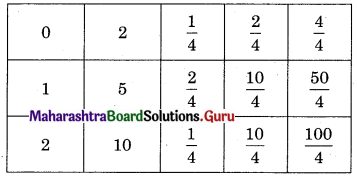Expected winning amount
E(Y) = Σpy = $$\frac{22}{4}$$ = ₹ 5.5
V(Y) = Σpy2 – (Σpy)2
= $$\frac{154}{4}$$ – (5.5)2
= 38.5 – 30.25
= ₹ 8.25Question 12.
Let the p.m.f. of the r.v. X be
$$p(x)= \begin{cases}\frac{3-x}{10} & \text { for } x=-1,0,1,2 \\ 0 & \text { otherwise }\end{cases}$$
Calculate E(X) and Var(X).
Solution: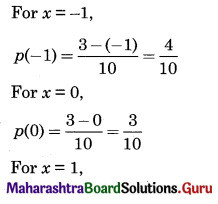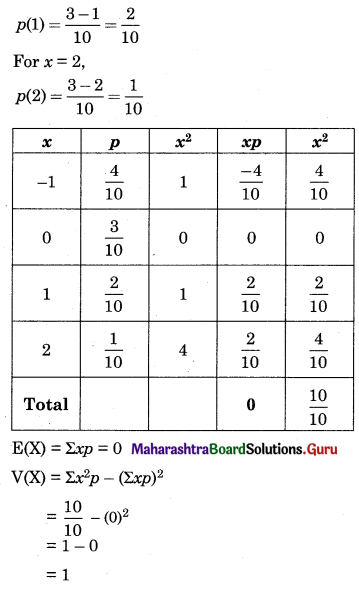Question 13.
Suppose error involved in making a certain measurement is a continuous r.v. X with p.d.f.
$$f(x)= \begin{cases}k\left(4-x^{2}\right) & \text { for }-2 \leq x \leq 2 \\ 0 & \text { otherwise }\end{cases}$$
Compute (i) P(X > 0), (ii) P(-1 < X < 1), (iii) P(X < -0.5 or X > 0.5)
Solution:
We know that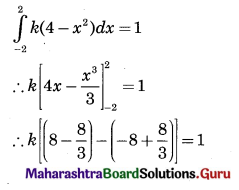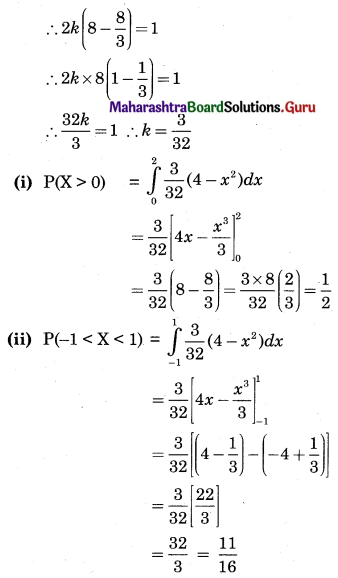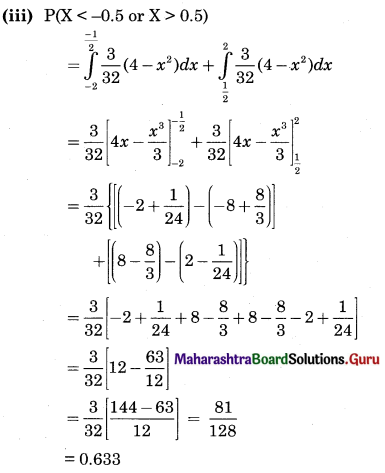Question 14.
The p.d.f. of the r.v. X is given by
$$f(x)= \begin{cases}\frac{1}{2 a} & \text { for } 0<x<2 a \\ 0 & \text { otherwise }\end{cases}$$
Show that P(X < $$\frac{a}{2}$$) = P(X > $$\frac{3a}{2}$$)
Solution: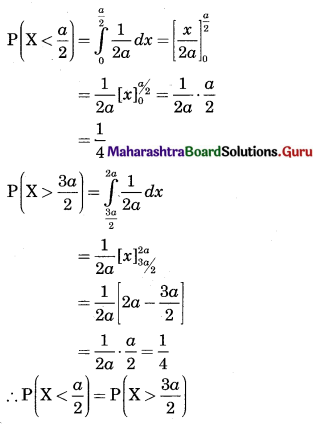Question 15.
Determine k if
$$f(x)= \begin{cases}k e^{-\theta x} & \text { for } 0 \leq x<\infty, \theta>0 \\ 0 & \text { otherwise }\end{cases}$$
is the p.d.f. of the r.v. X. Also find P(X > $$\frac{1}{\theta}$$). Find M if P(0 < X < M) = $$\frac{1}{2}$$
Solution:
We know that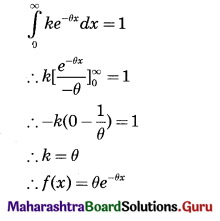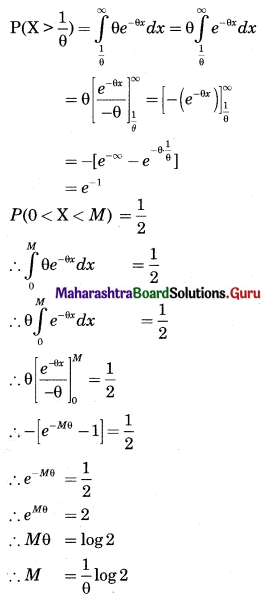Question 16.
The p.d.f. of the r.v. X is given by
$$f_{x}(x)=\left\{\begin{array}{l} \frac{k}{\sqrt{x}}, 0<x<4 \\ 0, \text { otherwise } \end{array}\right.$$
Determine k, c.d.f. of X and hence find P(X ≤ 2) and P(X ≥ 1).
Solution:
We know that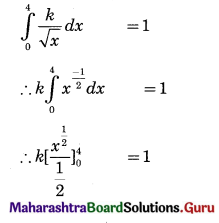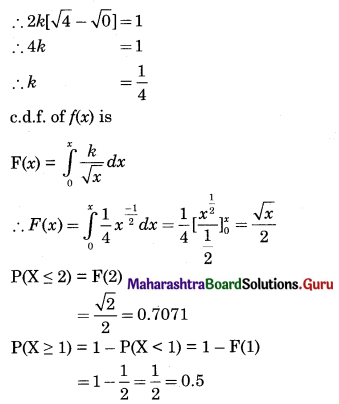Question 17.
Let X denote the reaction temperature (in °C) of a certain chemical process. Let X be a continuous r.v. with p.d.f.
$$f(x)= \begin{cases}\frac{1}{10}, & -5 \leq x \leq 5 \\ 0, & \text { otherwise }\end{cases}$$
Compute P(X < 0).
Solution:
Given p.d.f. is f(x) = $$\frac{1}{10}$$, for -5 ≤ x ≤ 5
Let its c.d.f. F(x) be given by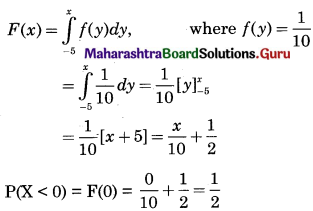Part – II

Question 1.
Let X ~ B(10, 0.2). Find (i) P(X = 1) (ii) P(X ≥ 1) (iii) P(X ≤ 8)
Solution:
X ~ B(10, 0.2)
n = 10, p = 0.2
∴ q = 1 – p = 1 – 0.2 = 0.8
(i) P(X = 1) = 10C1 (0.2)1 (0.8)9 = 0.2684

(ii) P(X ≥ 1) = 1 – P(X < 1)
= 1 – P(X = 0)
= 1 – 10C0 (0.2)0 (0.8)10
= 1 – 0.1074
= 0.8926

(iii) P(X ≤ 8) = 1 – P(x > 1)
= 1 – [p(9) + p(10)]
= 1 – [10C9 (0.2)9 (0.8)1 + 10C10 (0.2)10]
= 1 – 0.00000041984
= 0.9999Question 2.
Let X ~ B(n, p) (i) If n = 10 and E(X) = 5, find p and Var(X), (ii) If E(X) = 5 and Var(X) = 2.5, find n and p.
Solution:
X ~ B(n, p)
(i) n = 10, E(X) = 5
∴ np = 5
∴ 10p = 5
∴ p = $$\frac{1}{2}$$
∴ q = 1 – p = 1 – $$\frac{1}{2}$$ = $$\frac{1}{2}$$
V(X) = npq
= 10 × $$\frac{1}{2}$$ × $$\frac{1}{2}$$
= 2.5

(ii) E(X) = 5, V(X) = 2.5
∴ np = 5, ∴ npq = 2.5
∴ 5q = 2.5
∴ q = $$\frac{2.5}{5}$$ = 0.5, p = 1 – 0.5 = 0.5
But np = 5
∴ n(0.5) = 5
∴ n = 10

Question 3.
If a fair coin is tossed 4 times, find the probability that it shows (i) 3 heads, (ii) head in the first 2 tosses, and tail in the last 2 tosses.
Solution:
n : No. of times a coin is tossed
∴ n = 4
P : Probability of getting heads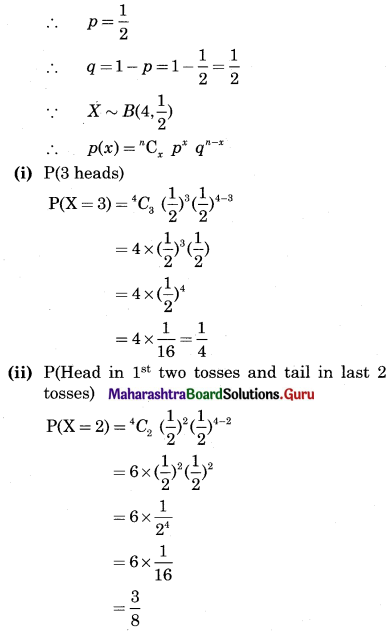Question 4.
The probability that a bomb will hit the target is 0.8. Find the probability that, out of 5 bombs, exactly 2 will miss the target.
Solution:
X : No. of bombs miss the target
p : Probability that bomb miss the target
∴ q = 0.8
∴ p = 1 – q = 1 – 0.8 = 0.2
n = No. of bombs = 5
∴ X ~ B(5, 0.2)
∴ p(x) = nCx px qn-x
P(X = 2) = 5C2 (0.2)2 (0.8)5-2
= 10 × 0.04 × (0.8)3
= 10 × 0.04 × 0.512
= 0.4 × 0.512
= 0.2048Question 5.
The probability that a lamp in the classroom will burn is 0.3. 3 lamps are fitted in the classroom. The classroom is unusable if the number of lamps burning in it is less than 2. Find the probability that the classroom can not be used on a random occasion.
Solution:
X : No. of lamps not burning
p : Probability that the lamp is not burning
∴ q = 0.3
∴ p = 1 – q = 1 – 0.3 = 0.7
n = No. of lamps fitted = 3
∴ X ~ B(3, 0.7)
∴ p(x) = nCx px qn-x
P(classroom cannot be used)
P(X < 2) = p(0) + p(1)
= 3C0 (0.7)0 (0.3)3-0 + 3C1 (0.7)1 (0.3)3-1
= 1 × 1 × (0.3)3 + 3 × 0.7 × (0.3)2
= (0.3)2 [0.3 + 3 × 0.7]
= 0.09 [0.3 + 2.1]
= 0.09 [2.4]
= 0.216

Question 6.
A large chain retailer purchases an electric device from the manufacturer. The manufacturer indicates that the defective rate of the device is 10%. The inspector of the retailer randomly selects 4 items from a shipment. Find the probability that the inspector finds at most one defective item in the 4 selected items.
Solution:
X : No. of defective items
n : No. of items selected = 4
p : Probability of getting defective items
∴ p = 0.1
∴ q = 1 – p = 1 – 0.1 = 0.9
P(At most one defective item)
P(X ≤ 1) = p(0) + p(1)
= 4C0 (0.1)0 (0.9)4-0 + 4C1 (0.1)1 (0.9)4-1
= 1 × 1 × (0.9)4 + 4 × 0.1 × (0.9)3
= (0.9)3 [0.9 + 4 × 0.1]
= (0.9)3 × [0.9 + 0.4]
= 0.729 × 1.3
= 0.9477

Question 7.
The probability that a component will survive a check test is 0.6. Find the probability that exactly 2 of the next 4 components tested survive.
Solution:
p = 0.6, q = 1 – 0.6 = 0.4, n = 4
x = 2
∴ p(x) = nCx px qn-x
P(X = 2) = 4C2 (0.6)2 (0.4)2 = 0.3456Question 8.
An examination consists of 5 multiple choice questions, in each of which the candidate has to decide which one of 4 suggested answers is correct. A completely unprepared student guesses each answer randomly. Find the probability that this student gets 4 or more correct answers.
Solution:
n : No. of multiple-choice questions
∴ n = 5
X : No. of correct answers
p : Probability of getting correct answer
∵ There are 4 options out of which one is correct
∴ p = $$\frac{1}{4}$$
∴ q = 1 – p = 1 – $$\frac{1}{4}$$ = $$\frac{3}{4}$$
∵ X ~ B(5, $$\frac{1}{4}$$)
∴ p(x) = nCx px qn-x
P(X ≥ 4) = p(4) + p(5)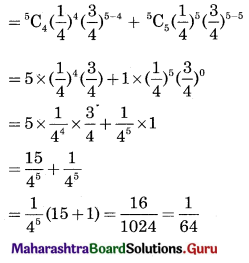Question 9.
The probability that a machine will produce all bolts in a production run with in the specification is 0.9. A sample of 3 machines is taken at random. Calculate the probability that all machines will produce all bolts in a production run within the specification.
Solution:
n : No. of samples selected
∴ n = 3
X : No. of bolts produce by machines
p : Probability of getting bolts
∴ p = 0.9
∴ q = 1 – p = 1 – 0.9 = 0.1
∴ X ~ B(3, 0.9)
∴ p(x) = nCx px qn-x
P(Machine will produce all bolts)
P(X = 3) = 3C3 (0.9)3 (0.1)3-3
= 1 × (0.9)3 × (0.1)0
= 1 × (0.9)3 × 1
= (0.9)3
= 0.729

Question 10.
A computer installation has 3 terminals. The probability that anyone terminal requires attention during a week is 0.1, independent of other terminals. Find the probabilities that (i) 0 (ii) 1 terminal requires attention during a week.
Solution:
n : No. of terminals
∴ n = 3
X : No. of terminals need attention
p : Probability of getting terminals need attention
∴ p = 0.1
∴ q = 1 – p = 1 – 0.1 = 0.9
∵ X ~ B(3, 0.1)
∴ p(x) = nCx px qn-x
(i) P(No attention)
∴ P(X = 0) = 3C0 × (0.1)0 (0.9)3-1
= 1 × 1 × (0.9)3
= 0.729

(ii) P(One terminal need attention)
∴ P(X = 1) = 3C1 (0.1)1 (0.9)3-1
= 3 × 0.1 × (0.9)2
= 0.3 × 0.81
= 0.243Question 11.
In a large school, 80% of the students like mathematics. A visitor asks each of 4 students, selected at random, whether they like mathematics, (i) Calculate the probabilities of obtaining an answer yes from all of the selected students, (ii) Find the probability that the visitor obtains the answer yes from at least 3 students.
Solution:
X : No. of students like mathematics
p: Probability that students like mathematics
∴ p = 0.8
∴ q = 1 – p = 1 – 0.8 = 0.2
n : No. of students selected
∴ n = 4
∵ X ~ B(4, 0.8)
∴ p(x) = nCx px qn-x
(i) P(All students like mathematics)
∴ P(X = 4) = 4C4 (0.8)4 (0.2)4-4
= 1 × (0.8)4 × (0.2)0
= 1 × (0.8)4 × 1
= 0.4096

(ii) P(Atleast 3 students like mathematics)
∴ P(X ≥ 3) = p(3) + p(4)
= 4C3 (0.8)3 (0.2)4-3 + 0.4096
= 4 × (0.8)3 (0.2)1 + 0.4096
= 0.8 × (0.8)3 + 0.4096
= (0.8)4 × 0.4096
= 0.4096 + 0.4096
= 0.8192

Question 12.
It is observed that it rains on 10 days out of 30 days. Find the probability that
(i) it rains on exactly 3 days of a week.
(ii) it rains at most 2 days a week.
Solution:
X : No. of days it rains in a week
p : Probability that it rains
∴ p = $$\frac{10}{30}=\frac{1}{3}$$
∴ q = 1 – p = 1 – $$\frac{1}{3}$$ = $$\frac{2}{3}$$
n : No. of days in a week
∴ n = 7
∴ X ~ B(7, $$\frac{1}{3}$$)
(i) P(Rains on Exactly 3 days of a week)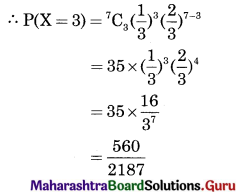(ii) P(Rains on at most 2 days of a week)
∴ P(X ≤ 2) = p(0) + p(1) + p(2)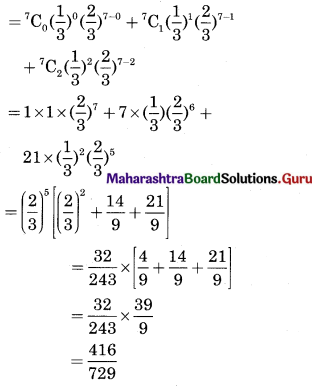Question 13.
If X follows Poisson distribution such that P(X = 1) = 0.4 and P(X = 2) = 0.2, find variance of X.
Solution:
X : Follows Possion Distribution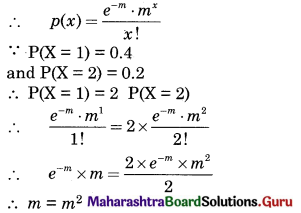∴ m = 1
∴ Mean = m = Variance of X = 1Question 14.
If X has Poisson distribution with parameter m, such that
$$\frac{P(X=x+1)}{P(X=x)}=\frac{m}{x+1}$$
find probabilities P(X = 1) and P(X = 2), when X follows Poisson distribution with m = 2 and P(X = 0) = 0.1353.
Solution:
Given that the random variable X follows the Poisson distribution with parameter m = 2
i.e. X ~ P(2)
Its p.m.f. is satisfying the given equation.
$$\frac{P(X=x+1)}{P(X=x)}=\frac{m}{x+1}$$
When x = 0,
$$\frac{\mathrm{P}(\mathrm{X}=1)}{\mathrm{P}(\mathrm{X}=0)}=\frac{2}{0+1}$$
P(X = 1) = 2P(X = 0)
= 2(0.1353)
= 0.2706
When x = 1,
$$\frac{\mathrm{P}(\mathrm{X}=2)}{\mathrm{P}(\mathrm{X}=1)}=\frac{2}{1+1}$$
P(X = 2) = P(X = 1) = 0.2706